Question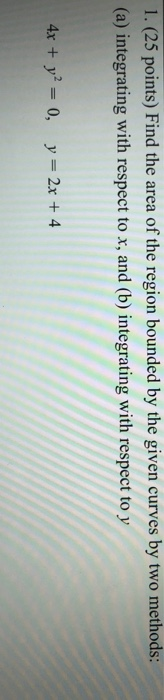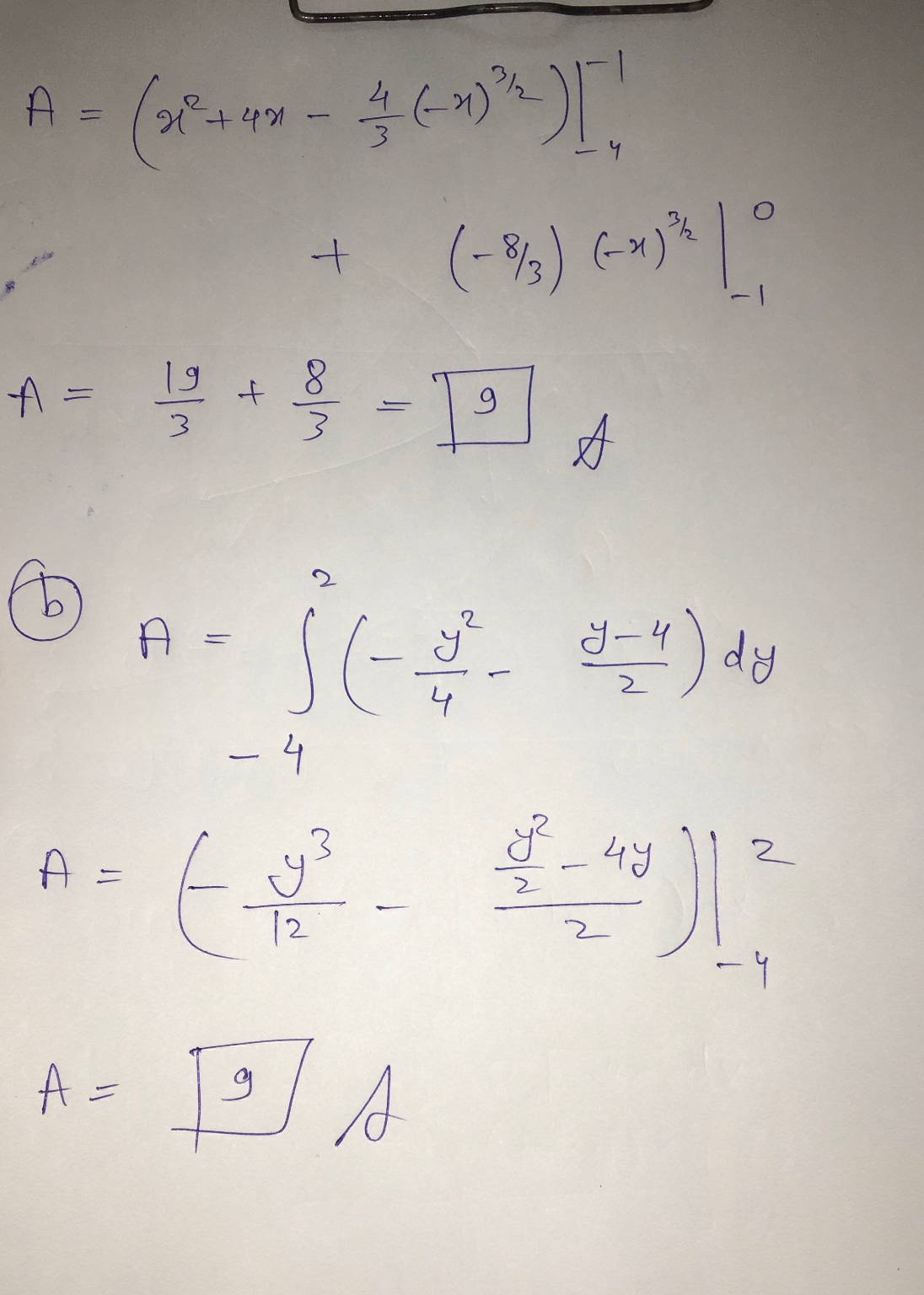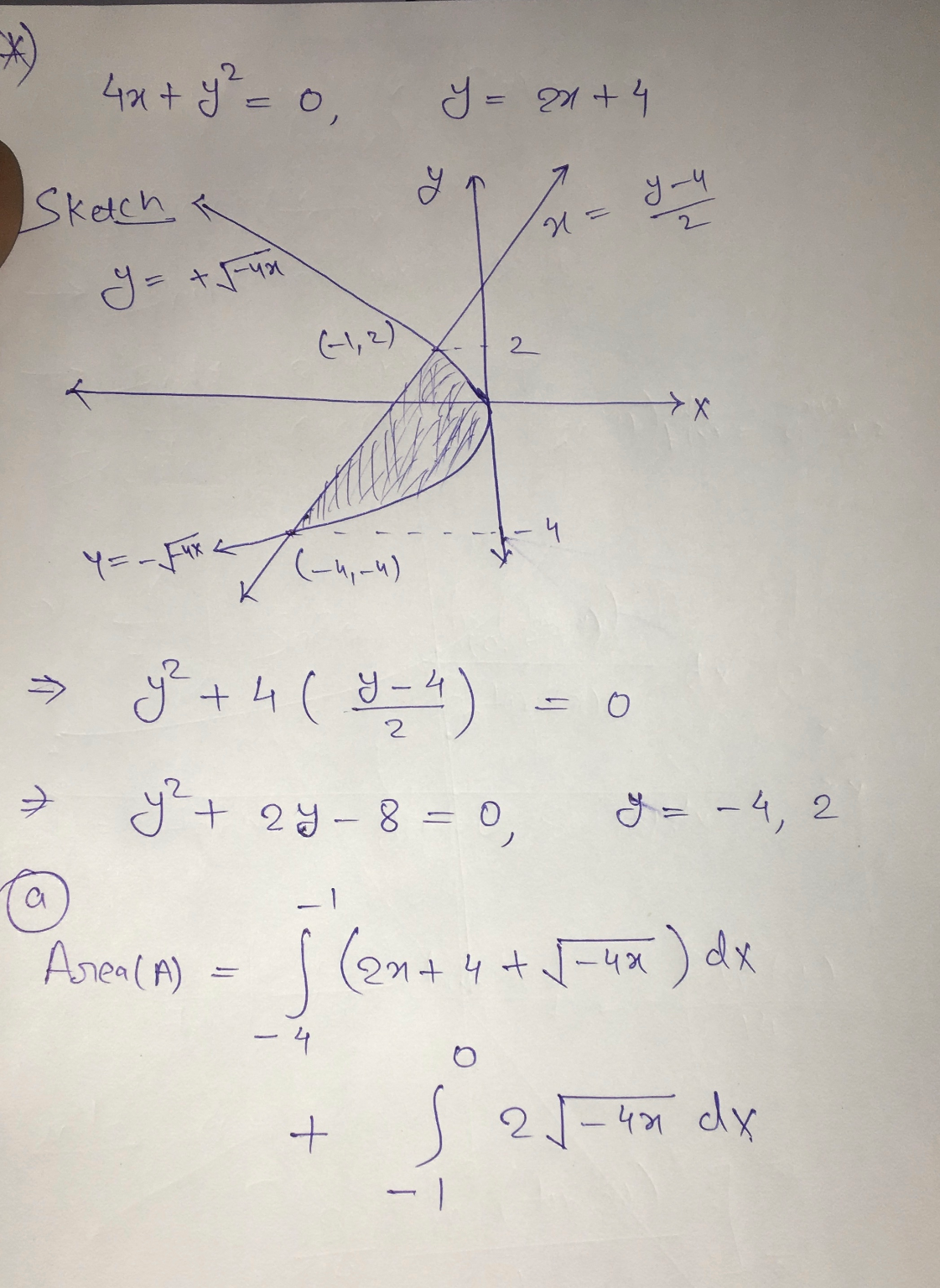#### Earn Coins

Coins can be redeemed for fabulous gifts.

Similar Homework Help Questions
• ### Find the area of the region bounded between the curves y = x and y =...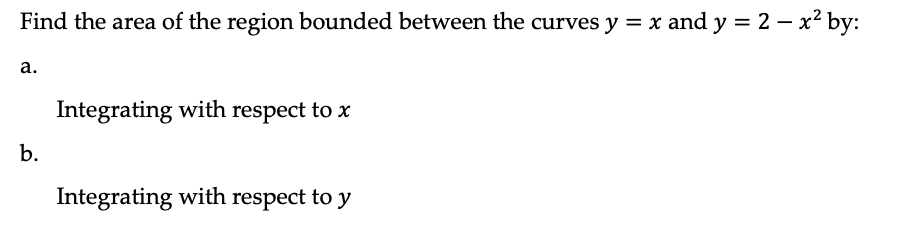Find the area of the region bounded between the curves y = x and y = 2 – x2 by: a. Integrating with respect to x Integrating with respect to y

• ### Find the area of the region between curves       1. Find Find the area of...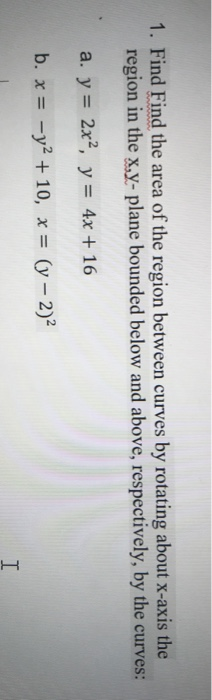Find the area of the region between curves       1. Find Find the area of the region between curves by rotating about x-axis the region in the x,y- plane bounded below and above, respectively, by the curves: a. y = 2x2, y = 4x + 16 b. x = -y2 + 10, x = (y – 2) I

• ### practice 1. Find the area of the region bounded by the curves. y= x2 - 4x,...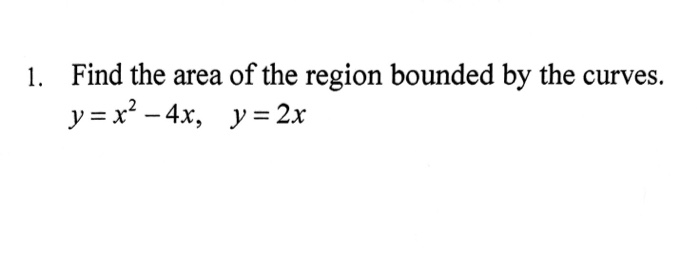practice 1. Find the area of the region bounded by the curves. y= x2 - 4x, y = 2x

• ### show all work 1. Find the area of the region bounded by the curves below. Sketch...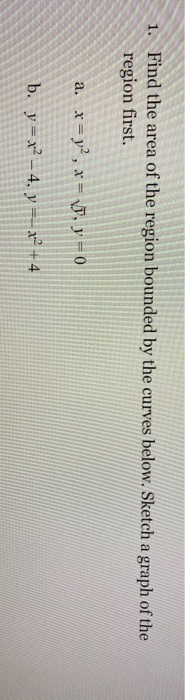show all work 1. Find the area of the region bounded by the curves below. Sketch a graph of the region first. a. x = y2, x = VD, y = 0 b. y = x2 – 4, y == x2 + 4

• ### Class Exercise 2. Find the volume generated by rotating the region bounded by the given curves...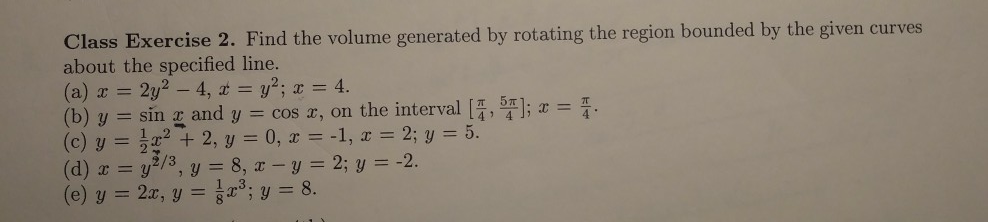Class Exercise 2. Find the volume generated by rotating the region bounded by the given curves about the specified line. 4, t y2; x = 4. 2y (b) y sin and y = cos x, on the interval [,]; x = . (c) y = 22, y 0, x -1 , x = 2; y 3/ 3, y = 8, x - y 2; y = -2. 5. (d) x = y (e) y = 2x, y =; y =...

• ### * 2. Find the area of the region bounded by the graphs of r = 3...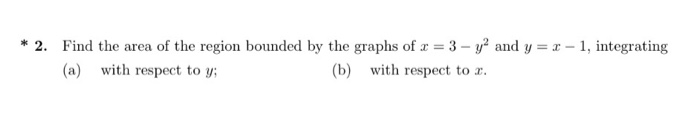* 2. Find the area of the region bounded by the graphs of r = 3 - y2 and y=r-1, integrating (a) with respect to y; (b) with respect to r.

• ### 16 pts) 1. Determine the area of the region between the two curves y=x and y+2x...16 pts) 1. Determine the area of the region between the two curves y=x and y+2x by integrating over the x-axis. Hint: Refer the figure and note that you will have two integrals to solve by splitting the region between the two curves into two smaller regions. lo pl [6 pts) 2. Find the area of the region bounded by the curves y=12 - x, y=vx, and y20

• ### Sketch the region enclosed by the given curves. Then, find its area by integrating with respect...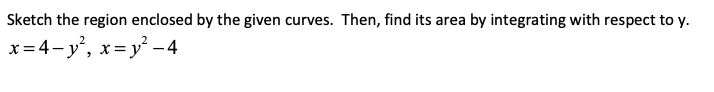Sketch the region enclosed by the given curves. Then, find its area by integrating with respect to y. x=4-y’, x= y² - 4

• ### Find the area of the region bounded by the two curves . y = x2 -...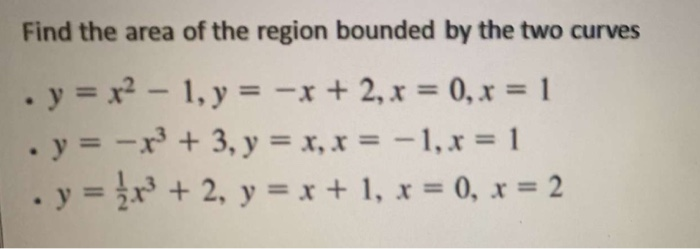Find the area of the region bounded by the two curves . y = x2 - 1, y = -x + 2, x = 0, x = 1 · y = -x + 3, y = x, x = -1, x = 1 . y = {x} + 2, y = x + 1, x = 0, x = 2

• ### (i) Find the area of the region bounded by the curves x = y 5y+6 and...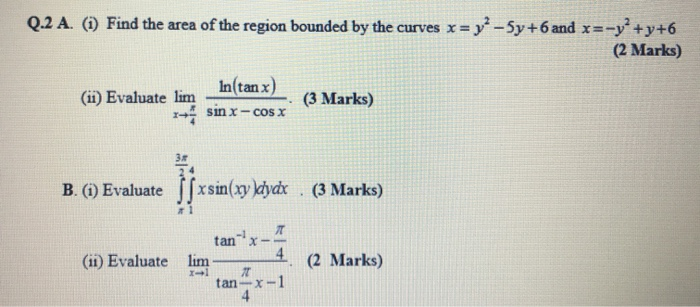(i) Find the area of the region bounded by the curves x = y 5y+6 and x =-y +y+6 Q.2 A. (1) Find the area of the region bounded by the curves x = y2 - 5y +6 and x=-y+y+6 (2 Marks) In(tan x) (ii) Evaluate lim (3 Marks) sinx-cosx B. (1) Evaluate |fxsin(xy dydx (3 Marks) X- (1) Evaluate lim * (11) Evaluate tan lim- (2 Marks) 2 Marks) - tan